# Acid base titration (1)

24 de Apr de 2015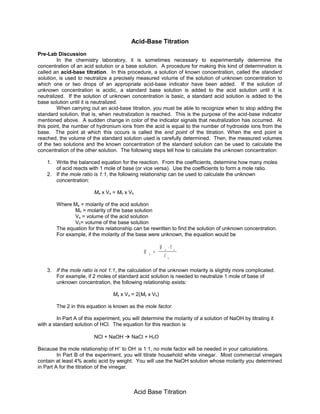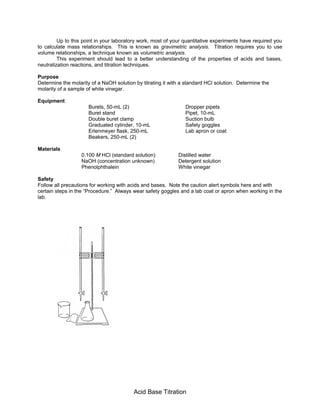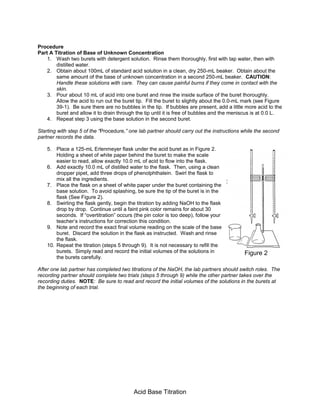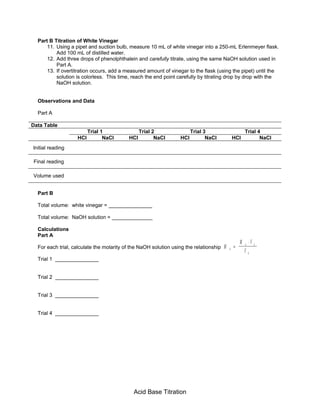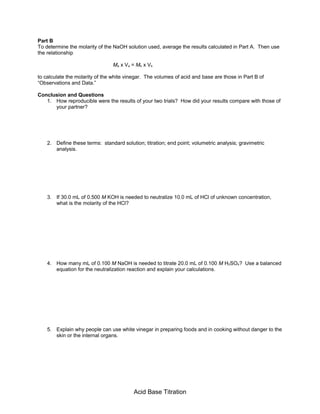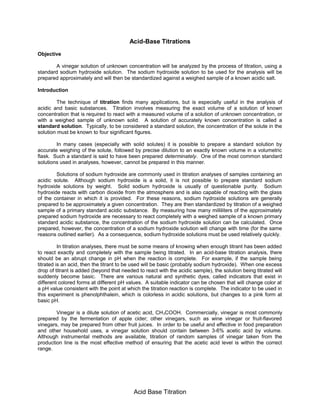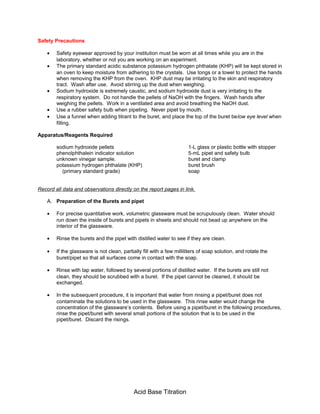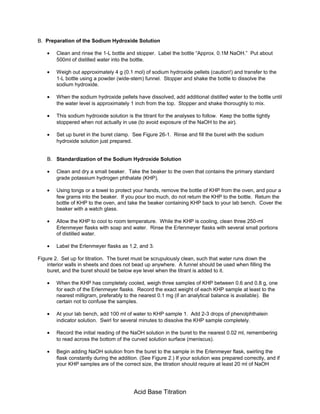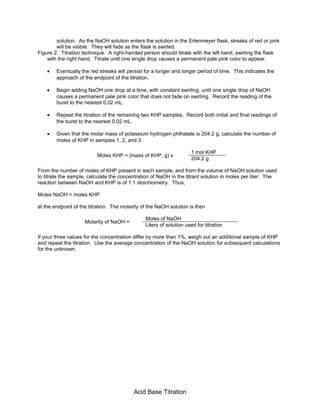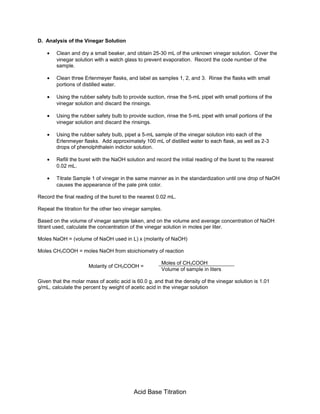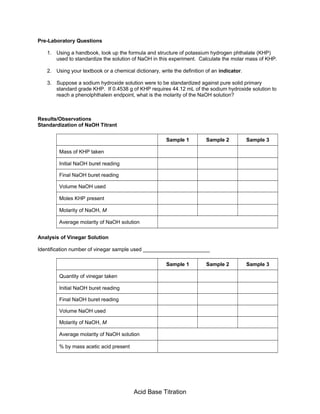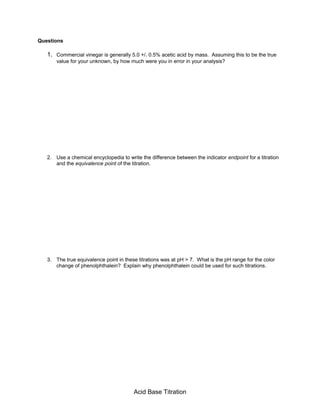Próximo SlideShare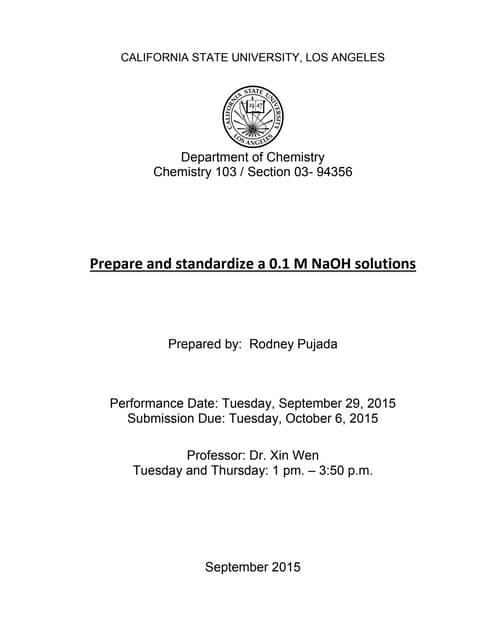Report 1 prepare and standardize a 0.1 M NaOH solutions
Cargando en ... 3
1 de 12

### Acid base titration (1)

1. Acid-Base Titration Pre-Lab Discussion In the chemistry laboratory, it is sometimes necessary to experimentally determine the concentration of an acid solution or a base solution. A procedure for making this kind of determination is called an acid-base titration. In this procedure, a solution of known concentration, called the standard solution, is used to neutralize a precisely measured volume of the solution of unknown concentration to which one or two drops of an appropriate acid-base indicator have been added. If the solution of unknown concentration is acidic, a standard base solution is added to the acid solution until it is neutralized. If the solution of unknown concentration is basic, a standard acid solution is added to the base solution until it is neutralized. When carrying out an acid-base titration, you must be able to recognize when to stop adding the standard solution, that is, when neutralization is reached. This is the purpose of the acid-base indicator mentioned above. A sudden change in color of the indicator signals that neutralization has occurred. At this point, the number of hydronium ions from the acid is equal to the number of hydroxide ions from the base. The point at which this occurs is called the end point of the titration. When the end point is reached, the volume of the standard solution used is carefully determined. Then, the measured volumes of the two solutions and the known concentration of the standard solution can be used to calculate the concentration of the other solution. The following steps tell how to calculate the unknown concentration: 1. Write the balanced equation for the reaction. From the coefficients, determine how many moles of acid reacts with 1 mole of base (or vice versa). Use the coefficients to form a mole ratio. 2. If the mole ratio is 1:1, the following relationship can be used to calculate the unknown concentration: Ma x Va = Mb x Vb Where Ma = molarity of the acid solution Mb = molarity of the base solution Va = volume of the acid solution Vb= volume of the base solution The equation for this relationship can be rewritten to find the solution of unknown concentration. For example, if the molarity of the base were unknown, the equation would be M M V Vb a a b = ⋅ 3. If the mole ratio is not 1:1, the calculation of the unknown molarity is slightly more complicated. For example, if 2 moles of standard acid solution is needed to neutralize 1 mole of base of unknown concentration, the following relationship exists: Ma x Va = 2(Mb x Vb) The 2 in this equation is known as the mole factor. In Part A of this experiment, you will determine the molarity of a solution of NaOH by titrating it with a standard solution of HCl. The equation for this reaction is NCl + NaOH  NaCl + H2O Because the mole relationship of H+ to OH- is 1:1, no mole factor will be needed in your calculations. In Part B of the experiment, you will titrate household white vinegar. Most commercial vinegars contain at least 4% acetic acid by weight. You will use the NaOH solution whose molarity you determined in Part A for the titration of the vinegar. Acid Base Titration
2. Up to this point in your laboratory work, most of your quantitative experiments have required you to calculate mass relationships. This is known as gravimetric analysis. Titration requires you to use volume relationships, a technique known as volumetric analysis. This experiment should lead to a better understanding of the properties of acids and bases, neutralization reactions, and titration techniques. Purpose Determine the molarity of a NaOH solution by titrating it with a standard HCl solution. Determine the molarity of a sample of white vinegar. Equipment Burets, 50-mL (2) Dropper pipets Buret stand Pipet, 10-mL Double buret clamp Suction bulb Graduated cylinder, 10-mL Safety goggles Erlenmeyer flask, 250-mL Lab apron or coat Beakers, 250-mL (2) Materials 0.100 M HCl (standard solution) Distilled water NaOH (concentration unknown) Detergent solution Phenolphthalein White vinegar Safety Follow all precautions for working with acids and bases. Note the caution alert symbols here and with certain steps in the “Procedure.” Always wear safety goggles and a lab coat or apron when working in the lab. Acid Base Titration